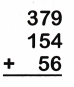Practice questions available in McGraw Hill Math Grade 2 Answer Key PDF Chapter 5 Lesson 3 Adding Three-Digit Numbers will engage students and is a great way of informal assessment.

Write the sum. Regroup if you need to.

Question 1.The sum of 611 and 208 is 819.

Question 2.Explanation:
The sum of 768 and 112 is 880.

Question 3.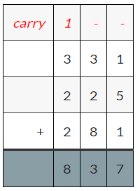Explanation:
The sum of 331, 225 and 281 is 837.

Question 4.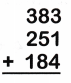Explanation:
The sum of 383, 251 and 184 is 818.

Question 5.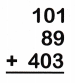Explanation:
The sum of 101, 89 and 403 is 593.

Question 6.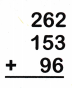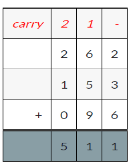Explanation:
The sum of 262, 153 and 96 is 511.

Question 7.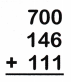Explanation:
The sum of 700, 146 and 111 is 957.

Question 8.